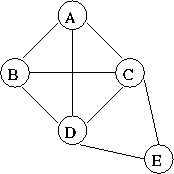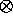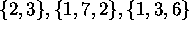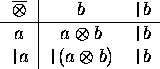# Special Cases of Parallel Prefix Networks, II

Tom Kelliher, CS 315

Mar. 31, 1999

### From Last Time

Began special cases.

### Outline

1. Parallel prefix on networks.

2. Parallel segmented prefix computations.

### Coming Up

Carry save, multiplication, convolution.

# Parallel Prefix on General Graphs

Recall idea: each vertex of the network simulates a leaf and an internal vertex of the tree.

Consider this example:1. Compute the breadth-first spanning tree from A.

2. Is the tree unique?

3. Run parallel prefix on the resulting tree.

# Segmented Prefix Computation

Definition:

A sequence of prefix computations that use the same associative operator, but on disjoint sets of data.

Example: Addition on.

1. Obviously, we can solve using three prefix computations. With a trick, we can do it in one.

2. The trick: use `|` as a barrier symbol at the start of each set of data.

3. The data set: `|`2, 3, `|`1, 7, 2, `|`1, 3, 6.

Redefine our operator:``Forget about everything to the left of the barrier.''

4. Run the example.

5. What is the running time of the parallel segmented prefix computation?

Thomas P. Kelliher
Wed Mar 31 10:08:15 EST 1999
Tom Kelliher